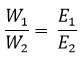## TyroCity

Chemistry 12 Notes for Chemistry Notes

Posted on • Updated on

# Determination Of Concentration Of Solution (Normality Equation)

After the determination of the volume, solution need to react completely at the equivalent point of reaction by using suitable indicator.
The concentration of given solution is determined by using normality.
The normality equation is obtained on the basis of law of chemical equivalent and the law of chemical equivalent states that, “the chemical substance react completely in the ratio of their equivalent masses.”Where, W1 and W2 are weight of reactants and E1 and E2 are their equivalent masses.
Or, x gram equivalent of A ≡ x gram equivalent of B
Or, 1 gram equivalent of A ≡ 1 gram equivalent of B
Or, 1000 ml of 1 N solution of A ≡ 1 gram equivalent of B
Or, 1000 ml of 1 N solution of A ≡ 1000 ml of 1 N solution of B
Or, V1 ml of S1 solution of A ≡ V2 ml of S2 N solution of B
V1S1 = V2S2 ——————– 1
Equation 1 is normality equation for titration and this expression is also called the basic principle of volumetric analysis. This is also working formula of titration.
V1 and V2 are the volume of solution needed to react completely at the equivalent point of reaction, and they are determined by titration by finding out the end point using useable indicator. S1 and S2 are normality factor of solution A and B.
To find the concentration of one of the solution using equation 1, the concentration of other must be known. And that solution whose concentration is known is standard solution. In titration, one of the solution must be standard solution.